# 0.PTA得分截图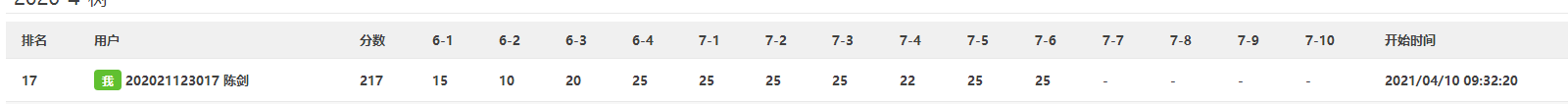# 1.本周学习总结（5分）

## 1.1 二叉树结构

### 1.1.1 二叉树的2种存储结构

#### 树的顺序存储结构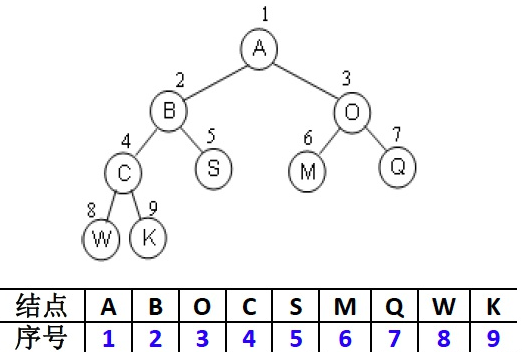#### 树的链式存储结构

typedef struct TreeNode *BinTree;
struct TreeNode{
ElementType Data;
BinTree Left;
BinTree Right;
}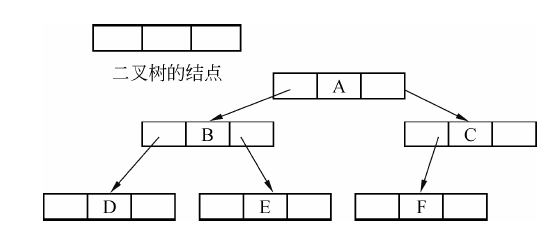### 1.1.2 二叉树的构造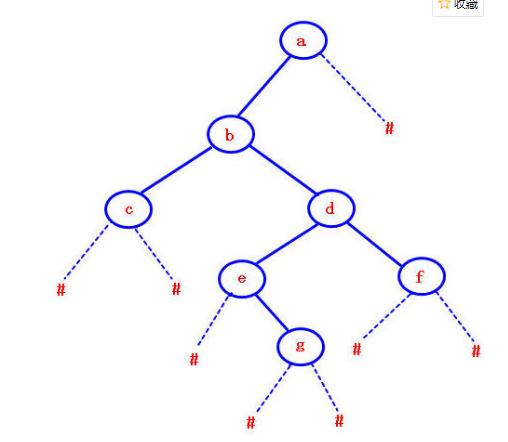#### 先序遍历序列

BTree CreatTree(char* str)//创建二叉树
{
if (str[i] == '#' || !str[i])
{
i++;
return NULL;
}
BTree T;
T = new TNode;
T->data = str[i++];
T->lchild = CreatTree(str);
T->rchild = CreatTree(str);
return T;
}


#### 后序遍历序列

• 如此便是前中后三种序列的构建，实际的应用中，只需更改下赋值跟递归的顺序，差别其实不大。

### 1.1.3 二叉树的遍历#### 先序遍历

（1）访问根结点。
（2）前序遍历左子树。
（3）前序遍历右子树 。

void preorder(BinTree BT)
{
if (!BT)
return;
if (!BT->Left && !BT->Right)
printf(" %c", BT->Data);
preorder(BT->Left);
preorder(BT->Right);
}


#### 中序遍历

（1）中序遍历左子树
（2）访问根结点
（3）中序遍历右子树

void inorder(BinTree BT)
{
if (!BT)
return;

inorder(BT->Left);
if (!BT->Left && !BT->Right)
printf(" %c", BT->Data);
inorder(BT->Right);
}


#### 后序遍历

（1）若二叉树为空，结束
(2）后序遍历左子树
(3）后序遍历右子树
(4）访问根结点

void postorder(BinTree BT)
{
if (!BT)
return;

postorder(BT->Left);
postorder(BT->Right);
if (!BT->Left && !BT->Right)
printf(" %c", BT->Data);
}


#### 层序遍历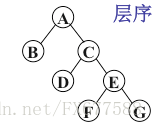A入队

void LevelTraversal(BinTree T)
{
if (!T)
{
cout << "NULL";
return;
}
BinTree p;
queue<BinTree>q;
q.push(T);
int flag = 1;
while (!(q.empty()))
{
p = q.front();//头结点
q.pop();//弹出头结点
if (flag)//访问结点
{
cout << p->Data;
flag = 0;
}
else if (!flag)
cout << " " << p->Data;
if (p->Left != NULL)
q.push(p->Left);
if (p->Right != NULL)
q.push(p->Right);
}
}


### 1.1.4 线索二叉树

#### 线索二叉树如何设计？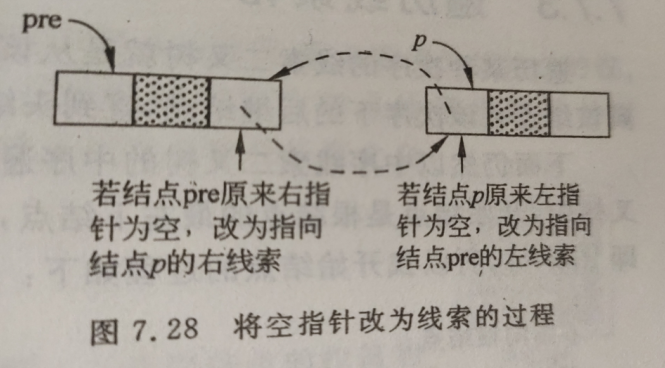iThrNodeType *pre;

BiThrTree InOrderThr(BiThrTree T)

{   /*中序遍历二叉树T，并将其中序线索化，pre为全局变量*/

pre->rtag=1;/*最后一个结点线索化*/

};

}

{/*通过中序遍历进行中序线索化*/

if(p)

if(p->lchild==NULL)/*前驱线索*/

{p->ltag=1;

p->lchild=pre;

}

if(p->lchild==NULL)/*后续线索*/

{p->rtag=1;

p->rchild=pre;

}

pre=p;

}

}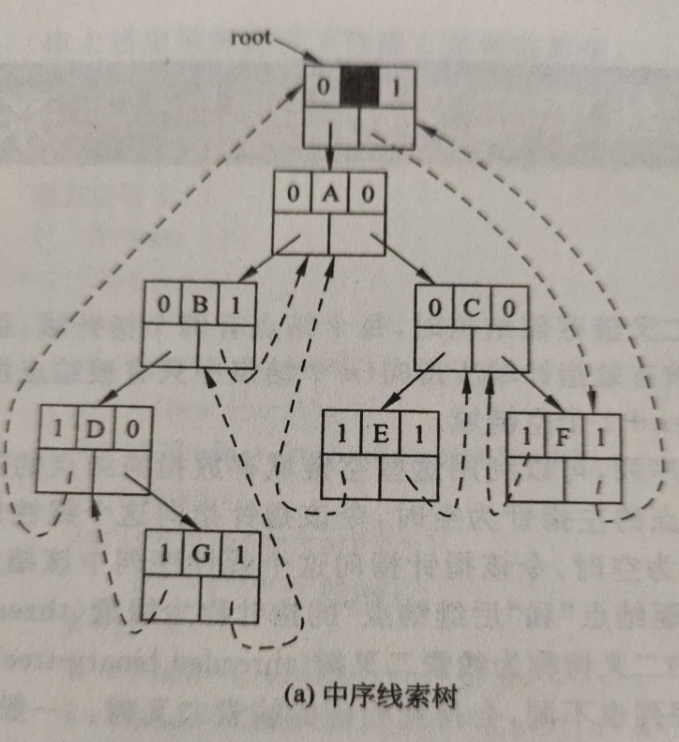### 1.1.5 二叉树的应用--表达式树

1. 依次读取表达式；
2. 如果是操作数，则将该操作数压入栈中；
3. 如果是操作符，则弹出栈中的两个操作数，第一个弹出的操作数作为右孩子，第二个弹出的操作数作为左孩子；然后再将该操作符压入栈中。
这样下去，就可以建立一颗完整的表达式树。

## 1.2 多叉树结构

### 1.2.1 多叉树结构

typedef struct node
{
char data;
struct node *sublist; //孩子链指针
}BTNode;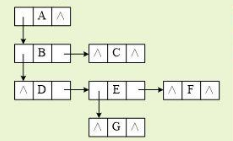### 1.2.2 多叉树遍历

1.访问根节点，
2.进行第一个孩子的优先的递归遍历，后进行后面其余孩子的递归遍历。

## 1.3 哈夫曼树

### 1.3.2 哈夫曼树构建及哈夫曼编码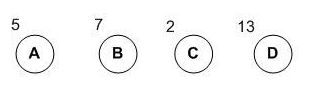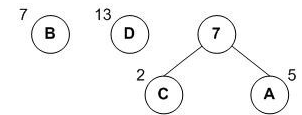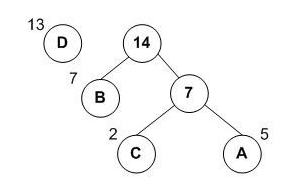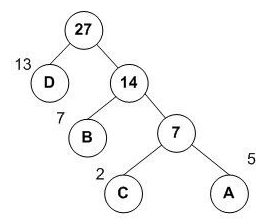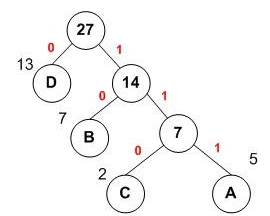A，B，C，D对应的哈夫曼编码分别为：111，10，110，0。

## 1.4 并查集

Find：确定元素属于哪一个子集。它可以被用来确定两个元素是否属于同一子集。
Union：将两个子集合并成同一个集合。

typedef struct node {
int data;              //结点对应人的编号
int rank;              //结点秩：子树的高度，合并用
int parent;            //结点对应双亲下标
} UFSTree;             //并查集树的结点类型


void MAKE_SET(UFSTree t[]，int n)//初始化并查集树
{
int i;
for(i=1;i<=n;i++)
{
t[i].data=0;  //数据为该人的编号
t[i].rank=0;  //秩初始化为0
t[i].parent=i;//双亲初始化指向自己
}


int FIND SET (UFSTree t[], int x) {    //在x所在的子树中查找集合编号
if (x!=t[x]. parent)                   //双亲不是自己
return(FIND-SET(t,t[x] . parent);      //递归在双亲中找x
else
return(x);                             //双亲是自己，返回x
}


void UNION(UFSTree t[], int x, int y)  //将x和y所在的子树合并
{
x=FIND-SET(t,x);                         //套找*所在分离集合树的编号
y=FIND SET(t,y);                         //查找y所在分离集合树的编号
if (t[x].rank > t[y].rank)               //y结点的秩小于x结点的秩
t[y].parent=x;                         //将y连到x结点上,*作为y的双亲结点
else                                     //y结点的秩大于等于x结点的科
{
t[x].parent=y;                           //将x连到y结点上,y作为x的双亲结点
if (t[x].rank==t[y]. rank)               //x和y结点的秩相同
t[y].rank++;                             //y结点的秩增
}
}


# 2.PTA实验作业（4分）

## 2.1 输出二叉树每层节点

### 2.1.1 解题思路及伪代码

if 该树为空
输出NULL

h记录层数,next 保存队列进队的下一层的层数,n为遍历的该层的层数
for h =1 to 队列不为空
输出h
while 队列不为空且i<n
出队一个树节点赋给p
if p为有数据的结点
输出p的值
然后进行p的左右孩子的出队并且next++
i++
End While
n = next 将下层结点的数保存的该层进行下一轮遍历
next =0
end for



# 3.阅读代码（0--1分）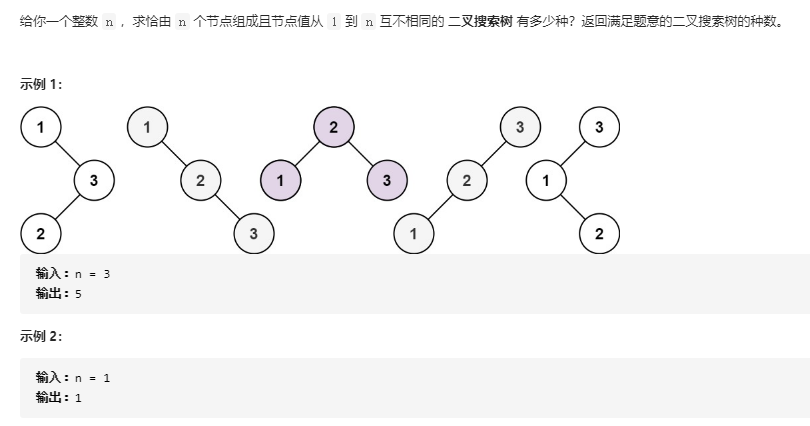3.1 不同的二叉搜索树

int numTrees(int n) {
int G[n + 1];
memset(G, 0, sizeof(G));
G = G = 1;
for (int i = 2; i <= n; ++i) {
for (int j = 1; j <= i; ++j) {
G[i] += G[j - 1] * G[i - j];
}
}
return G[n];
}



3.2 该题的设计思路及伪代码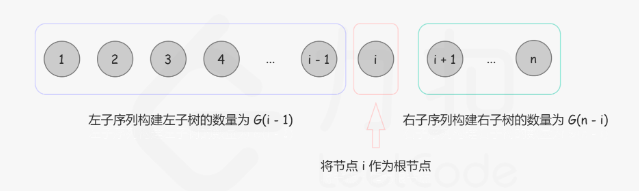G(n): 长度为 n 的序列能构成的不同二叉搜索树的个数。
F(i, n)F(i,n): 以 i 为根、序列长度为 n 的不同二叉搜索树个数 (1≤i≤n)。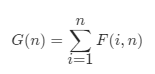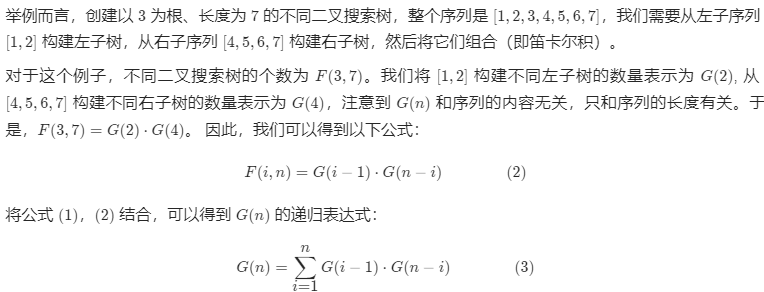定义数组G[]储存不同长度的搜索树的种类数量

for 2 to n
for 1 to i
根据公式计算G[I]的值3.3 分析该题目解题优势及难点。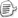posted on 2021-05-02 20:29    阅读(117)  评论(0编辑  收藏  举报